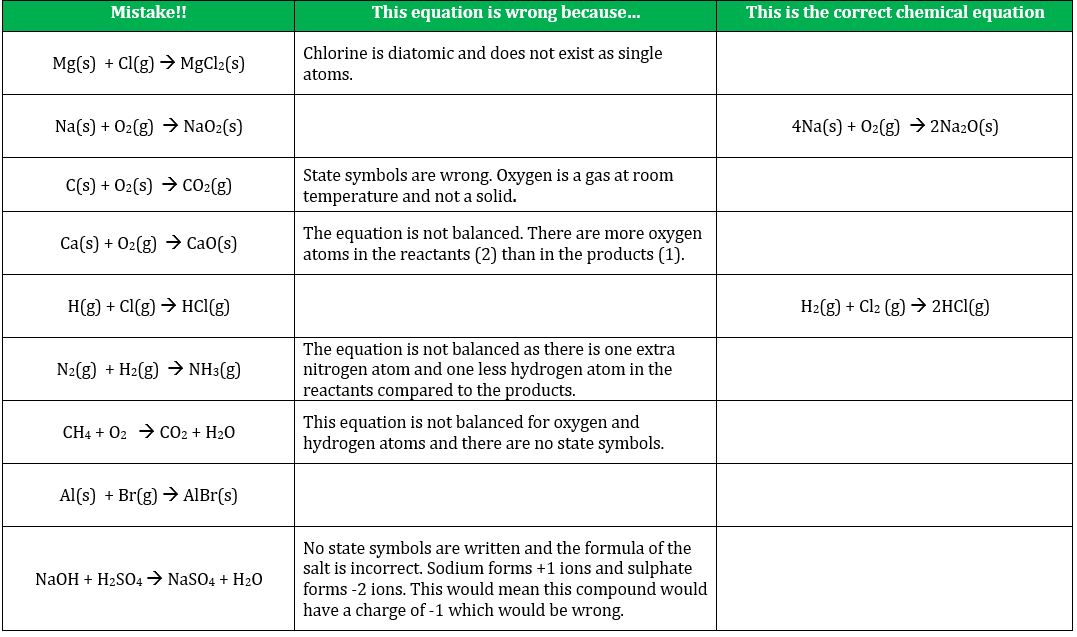# Balancing and writing chemical equations teaching resources

###### Worksheets and lesson ideas to challenge students aged 11 to 16 to think hard about balancing equations, conservation of mass, writing chemical equations and formula. (GCSE and Key Stage 3)
Balancing and writing chemical equations teacher brief

Overview: when substances take part in a chemical reaction, atoms or ions are rearranged. However, the total number of each type of atom (or ion) must remain the same – we cannot create or destroy atoms in a chemical reaction, we can simply change what they are joined to. To understand chemistry, students must be confident in representing chemical and physical changes with chemical equations, and this requires lots of practice and exposure. Students also need to understand where the mass of an atom comes from, i.e. the nucleus.

Key concept: atoms cannot be created or destroyed in a chemical reaction, they can simply change what they are joined to

Linked knowledge: states of matter; bonding; chemical changes; atomic structure

Misconception/error [scientific idea]: when you balance an equation you can change the formula of a substance [you can only change how many of each substance we have]; simple covalent elements e.g. chlorine and hydrogen are monatomic [simple covalent elements are diatomic]; ionic compounds have molecular formulas [only covalent substances have molecular formulas]

## Teaching resources

#### Where to start?

A great starting point is to ask students what they think will happen to the mass of iron wool when it is burnt in air. Many will incorrectly say the mass will decrease (or they may argue mass will remain the same, quoting law of conservation). Record the mass of some iron wool using a top pan balance. Then, using tongs, heat the wool in a Bunsen flame. Record the new mass. Ask students to explain the apparent increase in mass. How is this possible? Write a simple equation to represent this reaction and then show students why we need to balance an equation and add state symbols. A nice set-up from the RSC is here. You could represent this equation using molymods too.

#### Conservation of mass

Key Stage 3 activity on conservation of mass Students use a formative multiple choice question to explore their thinking of conservation of mass following a demonstration. Students then consider why some reactions appear not to support the law of conservation before they have to refute the misconception. (PDF)

#### Chemical formulae

Resources to teach chemical formulae are here.

#### How to balance chemical equations

GCSE and Key Stage 3 activity on how to balance chemical equations. Students balance simple equations involving concrete examples, such as wheels and cars, by adding coefficients before the object. This activity helps make the jump to more abstract chemical equations easier. Try it before you balance chemical equations – it will hopefully help students understand why we need to balance equations in the first place. (PDF)

#### Why do we need to balance equations?

This video is an excellent introduction to the importance of stoichiometry when reacting substances. A sufficient BANG is only produced when one part oxygen is mixed with two parts hydrogen. Students must have a good understanding of why we need to balance chemical equations before we introduce coefficients and mole ratios.

GCSE activity on conservation of mass using the hydrogen balloon demonstration. Students think about why chemists balance equations. The activity uses the combustion of hydrogen as an example and links to the video above. Combustion of hydrogen gas is an engaging demonstration that will provide the ‘hook’ to introduce the more abstract concept of balancing and writing equations. (PDF)

#### Writing chemical equations: state symbols and balancingGCSE worksheet on writing chemical equations. It is absolutely vital that students are able to understand the language of chemical equations if they are going to enjoy and thrive in this subject. The purpose of this exercise is to diagnose whether students can write chemical equation to enable feedback to take place. (PDF)

#### Writing ionic equations for precipitation reactions

GCSE worksheet on ionic equations for precipitation reactions. This activity has been designed to help students understand why we use ionic equations in chemistry. It is important that students are fluent with stating the formula and charges of common ions before they complete this task. (PDF)

#### Going deeper

• The human population on Earth is increasing year on year. What is happening to the mass of the Earth?
• Does 1 gram of sodium and 1 gram of neon have the same number of atoms?
• What would happen to the mass of a piece of magnesium if it was burnt in air? Use a balanced symbol equation to explain your answer. Why does this not contradict the law of conservation of mass?
• Why do chemists use empirical formulas?

Top